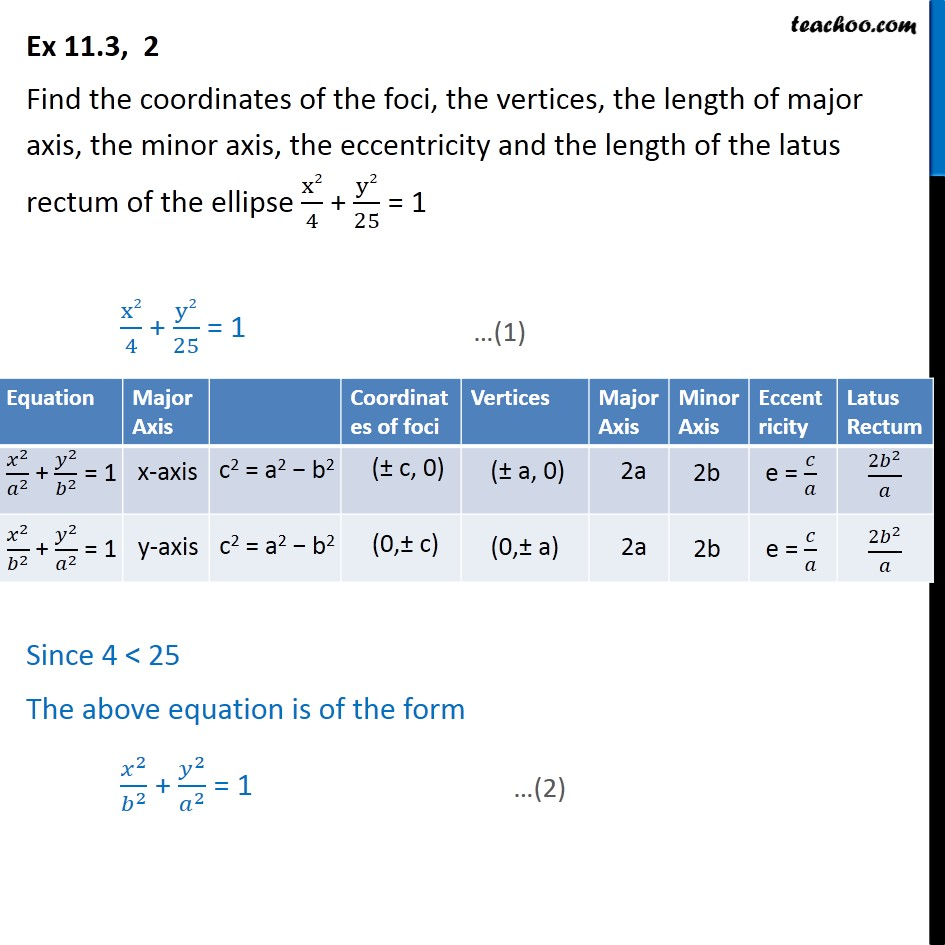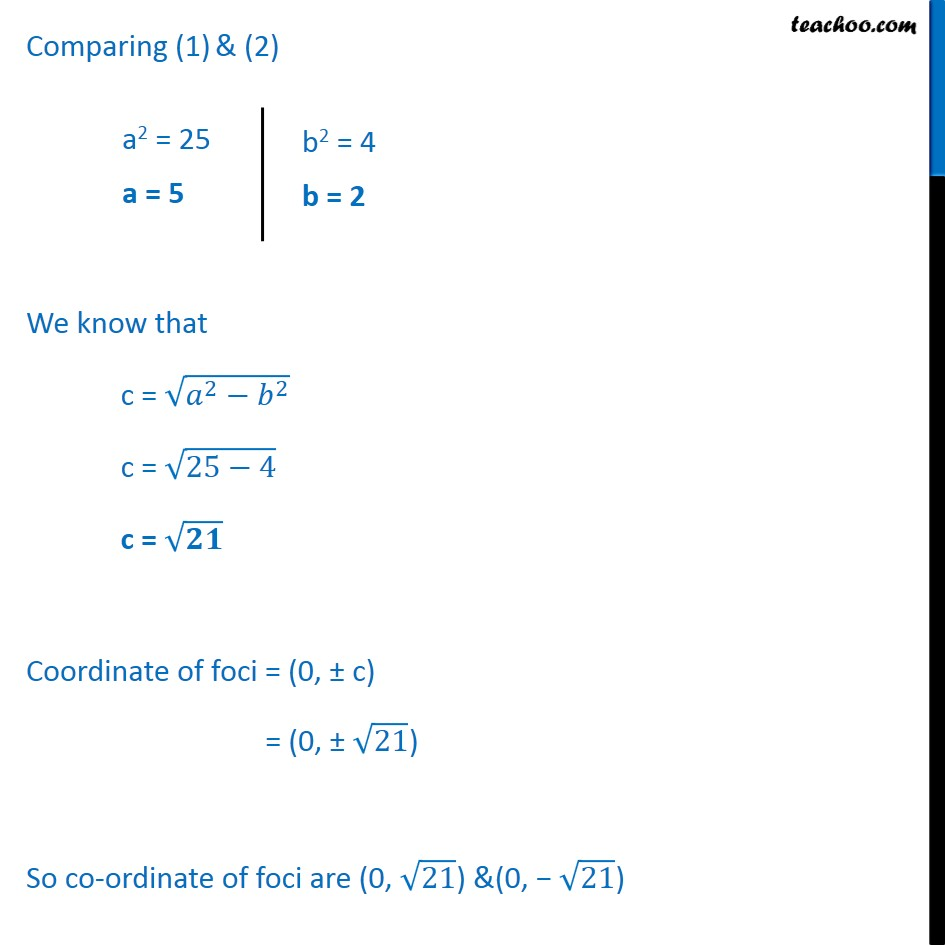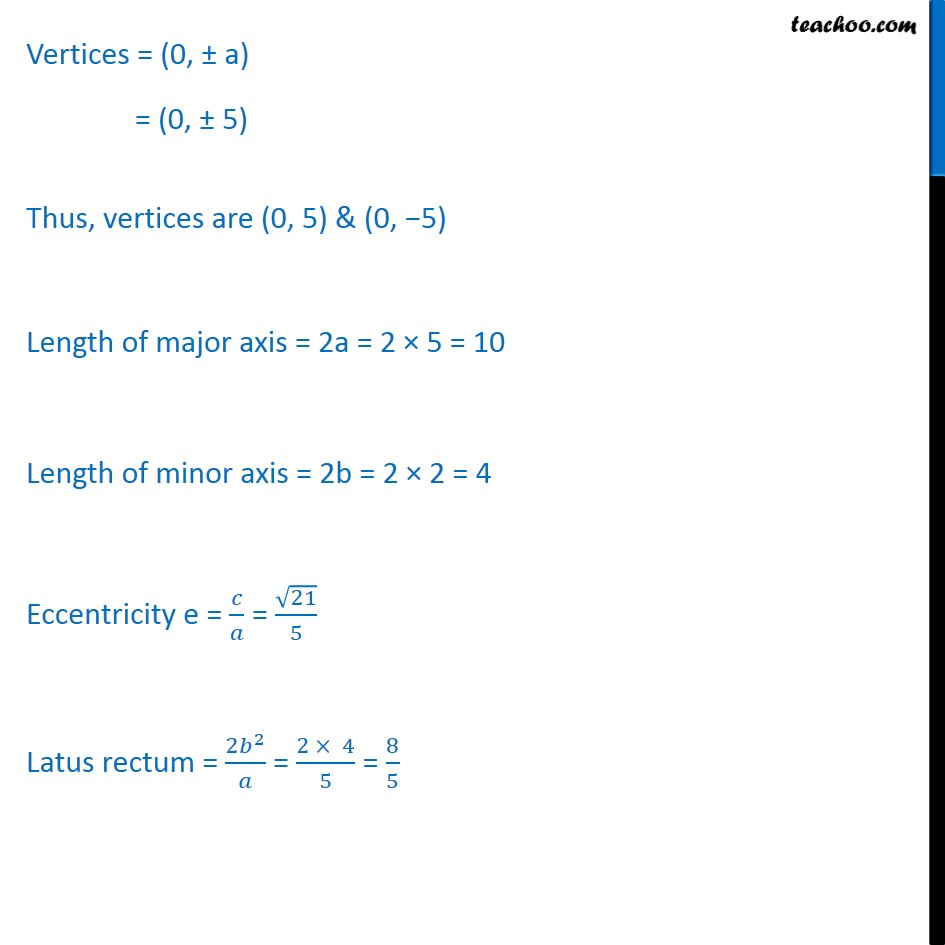Ex 10.3

Chapter 10 Class 11 Conic Sections
Serial order wiseLearn in your speed, with individual attention - Teachoo Maths 1-on-1 Class

### Transcript

Ex 10.3, 2 Find the coordinates of the foci, the vertices, the length of major axis, the minor axis, the eccentricity and the length of the latus rectum of the ellipse ﷐x2﷮4﷯ + ﷐y2﷮25﷯ = 1 ﷐x2﷮4﷯ + ﷐y2﷮25﷯ = 1 Since 4 < 25 The above equation is of the form ﷐﷐𝑥﷮2﷯﷮﷐𝑏﷮2﷯﷯ + ﷐﷐𝑦﷮2﷯﷮﷐𝑎﷮2﷯﷯ = 1 Comparing (1) & (2) We know that c = ﷐﷮﷐𝑎﷮2﷯−﷐𝑏﷮2﷯﷯ c = ﷐﷮25−4﷯ c = ﷐﷮𝟐𝟏﷯ Coordinate of foci = (0, ± c) = (0, ± ﷐﷮21﷯) So co-ordinate of foci are (0, ﷐﷮21﷯) &(0, − ﷐﷮21﷯) Vertices = (0, ± a) = (0, ± 5) Thus, vertices are (0, 5) & (0, −5) Length of major axis = 2a = 2 × 5 = 10 Length of minor axis = 2b = 2 × 2 = 4 Eccentricity e = ﷐𝑐﷮𝑎﷯ = ﷐﷐﷮21﷯﷮5﷯ Latus rectum = ﷐2﷐𝑏﷮2﷯﷮𝑎﷯ = ﷐2 × 4﷮5﷯ = ﷐8﷮5﷯